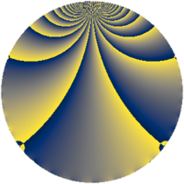# Properties

 Label 1140.2.mLevel $1140$ Weight $2$ Character orbit 1140.m Rep. character $\chi_{1140}(379,\cdot)$ Character field $\Q$ Dimension $120$ Sturm bound $480$

# Related objects

## Defining parameters

 Level: $$N$$ $$=$$ $$1140 = 2^{2} \cdot 3 \cdot 5 \cdot 19$$ Weight: $$k$$ $$=$$ $$2$$ Character orbit: $$[\chi]$$ $$=$$ 1140.m (of order $$2$$ and degree $$1$$) Character conductor: $$\operatorname{cond}(\chi)$$ $$=$$ $$380$$ Character field: $$\Q$$ Sturm bound: $$480$$

## Dimensions

The following table gives the dimensions of various subspaces of $$M_{2}(1140, [\chi])$$.

Total New Old
Modular forms 248 120 128
Cusp forms 232 120 112
Eisenstein series 16 0 16

## Trace form

 $$120q - 4q^{4} - 4q^{6} - 120q^{9} + O(q^{10})$$ $$120q - 4q^{4} - 4q^{6} - 120q^{9} + 4q^{16} - 8q^{20} + 4q^{24} - 40q^{26} + 4q^{36} - 56q^{44} + 136q^{49} + 4q^{54} - 32q^{61} - 52q^{64} + 24q^{66} - 32q^{74} - 28q^{76} + 24q^{80} + 120q^{81} - 40q^{85} - 44q^{96} + O(q^{100})$$

## Decomposition of $$S_{2}^{\mathrm{new}}(1140, [\chi])$$ into newform subspaces

The newforms in this space have not yet been added to the LMFDB.

## Decomposition of $$S_{2}^{\mathrm{old}}(1140, [\chi])$$ into lower level spaces

$$S_{2}^{\mathrm{old}}(1140, [\chi]) \cong$$ $$S_{2}^{\mathrm{new}}(380, [\chi])$$$$^{\oplus 2}$$